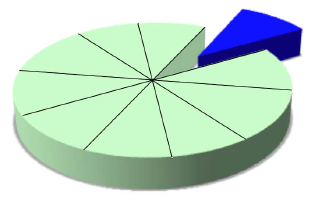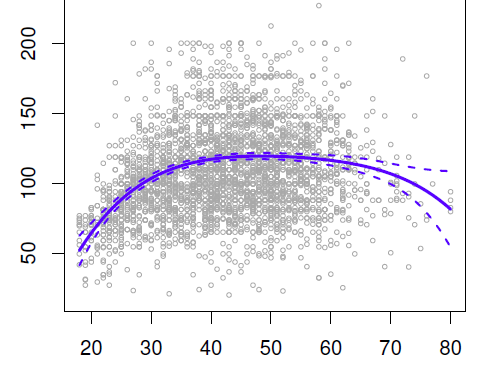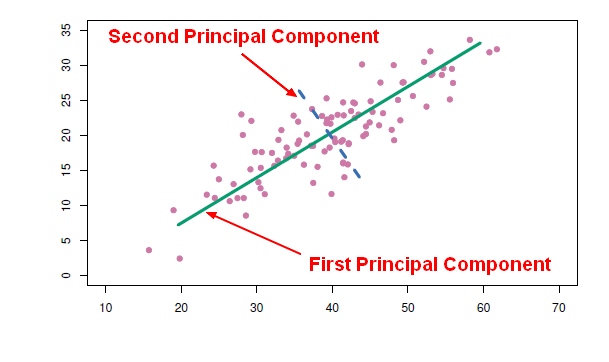# Statistics - Bias (Sampling error)

Bias in stats

Bias is a systematic error in sampling or measurement.

Systematic measurement error represents bias.

It has an effect on the entire distribution (It shift it right or left).

The bias is how far off on the average the model is from the truth.

More the model is complex, more the bias will decrease.

## Example

• Measurement Bias: An instrument that will always measure with the same difference.
• Sampling Bias: If we made our sample in an area where a large number of person tend to congregate (such as a city), it may lead to population estimate that is too high.

Recommended Pages(Statistics|Data Mining) - (K-Fold) Cross-validation (rotation estimation)

Cross-validation, sometimes called rotation estimation is a resampling validation technique for assessing how the results of a statistical analysis will generalize to an independent new data set. This...Data Mining - (Test|Expected|Generalization) Error

Test error is the prediction error that we incur on new data. The test error is actually how well we'll do on future data the model hasn't seen. The test error is the average error that results from using...Data Mining - (Function|Model)

The model is the function, equation, algorithm that predicts an outcome value from one of several predictors. During the training process, the models are build. A model uses a logic and one of several...Data Mining - (Global) Polynomial Regression (Degree)

polynomials regression Although polynomials are easy to think of, splines are much better behaved and more local. With polynomial regression, you create new variables that are just transformations...Data Mining - Decision boundary Visualization

Classifiers create boundaries in instance space. Different classifiers have different biases. You can explore them by visualizing the classification boundaries. Logistic Regression method produces...Data Mining - Noise (Unwanted variation)

Information from all past experience can be divided into two groups: information that is relevant for the future (“signal”) information that is irrelevant (“noise”). In many cases the factors...Data Mining - Principal Component (Analysis|Regression) (PCA|PCR)

Principal Component Analysis (PCA) is a feature extraction method that use orthogonal linear projections to capture the underlying variance of the data. By far, the most famous dimension reduction approach...Data Mining - Random forest

Random forest (or random forests) is a trademark term for an ensemble classifier that consists of many decision trees and outputs the class that is the mode of the classes output by individual trees. ...Machine Learning - (Overfitting|Overtraining|Robust|Generalization) (Underfitting)

A learning algorithm is said to overfit if it is: more accurate in fitting known data (ie training data) (hindsight) but less accurate in predicting new data (ie test data) (foresight) Ie the model...Model Building - ReSampling Validation

Resampling method are a class of methods that estimate the test error by holding out a subset of the training set from the fitting process, and then applying the statistical learning method to those held...